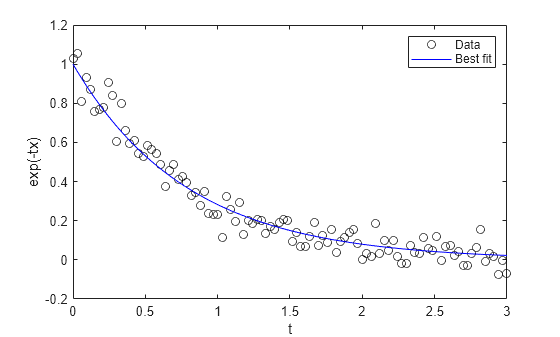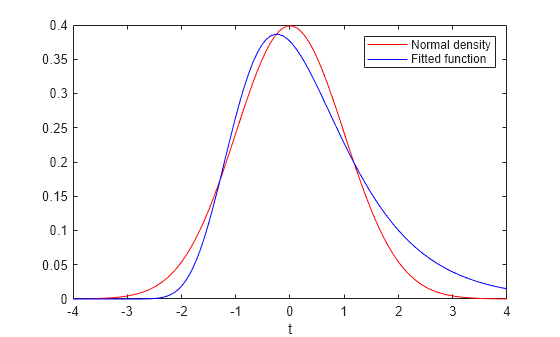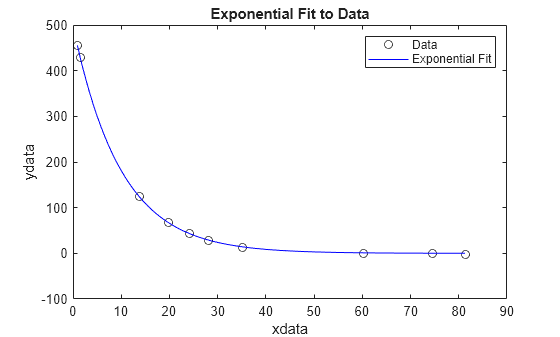# lsqnonlin

## 语法

``x = lsqnonlin(fun,x0)``
``x = lsqnonlin(fun,x0,lb,ub)``
``x = lsqnonlin(fun,x0,lb,ub,A,b,Aeq,beq)``
``x = lsqnonlin(fun,x0,lb,ub,A,b,Aeq,beq,nonlcon)``
``x = lsqnonlin(fun,x0,lb,ub,options)``
``x = lsqnonlin(fun,x0,lb,ub,A,b,Aeq,beq,nonlcon,options)``
``x = lsqnonlin(problem)``
``[x,resnorm] = lsqnonlin(___)``
``[x,resnorm,residual,exitflag,output] = lsqnonlin(___)``
``[x,resnorm,residual,exitflag,output,lambda,jacobian] = lsqnonlin(___)``

## 说明

`$\underset{x}{\mathrm{min}}{‖f\left(x\right)‖}_{2}^{2}=\underset{x}{\mathrm{min}}\left({f}_{1}{\left(x\right)}^{2}+{f}_{2}{\left(x\right)}^{2}+...+{f}_{n}{\left(x\right)}^{2}\right)$`

`$\begin{array}{c}\text{lb}\le x\\ x\le \text{ub}\\ Ax\le b\\ \text{Aeq}x=\text{beq}\\ c\left(x\right)\le 0\\ \text{ceq}\left(x\right)=0.\end{array}$`

x、`lb``ub` 可以是向量或矩阵；请参阅矩阵参数

`$f\left(x\right)=\left[\begin{array}{c}{f}_{1}\left(x\right)\\ {f}_{2}\left(x\right)\\ ⋮\\ {f}_{n}\left(x\right)\end{array}\right].$`

````x = lsqnonlin(fun,x0)` 从点 `x0` 开始，求 `fun` 中所描述函数的平方和的最小值。函数 `fun` 应返回由值（而不是值的平方和）组成的向量（或数组）。（该算法隐式计算 `fun(x)` 的分量的平方和。） 注意传递额外参数说明如何在必要时为向量函数 `fun(x)` 传递额外参数。 ```

````x = lsqnonlin(fun,x0,lb,ub)` 对 `x` 中的设计变量定义一组下界和上界，使解始终在 `lb `≤` x `≤` ub` 范围内。您可以通过指定 `lb(i) = ub(i)` 来修复解分量 `x(i)`。 注意如果问题的指定输入边界不一致，则输出 `x` 为 `x0`，输出 `resnorm` 和 `residual` 为 `[]`。违反边界 `lb ≤ x ≤ ub` 的 `x0` 的分量将重置为位于由边界定义的框内。遵守边界的分量不会更改。 ```

``x = lsqnonlin(fun,x0,lb,ub,A,b,Aeq,beq)` 要求解满足以下线性约束A x ≤ bAeq x = beq.`

``x = lsqnonlin(fun,x0,lb,ub,A,b,Aeq,beq,nonlcon)` 要求解满足 `nonlcon`(`x`) 函数中的非线性约束。`nonlcon` 返回两个输出，即 `c` 和 `ceq`。求解器尝试满足以下约束c ≤ 0`ceq` = 0.`

``x = lsqnonlin(fun,x0,lb,ub,options)` 和 `x = lsqnonlin(fun,x0,lb,ub,A,b,Aeq,beq,nonlcon,options)` 使用 `options` 中指定的优化选项执行最小化。使用 `optimoptions` 可设置这些选项。如果参数不存在，则为 `lb` 和 `ub` 及其他输入参数传递空矩阵。`
``x = lsqnonlin(problem)` 求 `problem` 的最小值，它是 `problem` 中所述的一个结构体。`

`对于任何输入参数，`[x,resnorm] = lsqnonlin(___)` 返回在 `x` 处的残差的 2-范数平方值：`sum(fun(x).^2)`。`

``[x,resnorm,residual,exitflag,output] = lsqnonlin(___)` 还返回在解 `x` 处的残差 `fun(x)` 的值、描述退出条件的值 `exitflag`，以及包含优化过程信息的结构体 `output`。`
``[x,resnorm,residual,exitflag,output,lambda,jacobian] = lsqnonlin(___)` 还返回结构体 `lambda`（其字段包含在解 `x` 处的拉格朗日乘数），以及 `fun` 在解 `x` 处的雅可比矩阵。`

## 示例

`$y=\mathrm{exp}\left(-1.3t\right)+\epsilon ,$`

```rng default % for reproducibility d = linspace(0,3); y = exp(-1.3*d) + 0.05*randn(size(d));```

`fun = @(r)exp(-d*r)-y;`

```x0 = 4; x = lsqnonlin(fun,x0)```
```Local minimum possible. lsqnonlin stopped because the final change in the sum of squares relative to its initial value is less than the value of the function tolerance. ```
```x = 1.2645 ```

```plot(d,y,'ko',d,exp(-x*d),'b-') legend('Data','Best fit') xlabel('t') ylabel('exp(-tx)')````$a\mathrm{exp}\left(-t\right)\mathrm{exp}\left(-\mathrm{exp}\left(-\left(t-b\right)\right)\right)$`

`$\frac{1}{\sqrt{2\pi }}\mathrm{exp}\left(-{t}^{2}/2\right).$`

```t = linspace(-4,4); y = 1/sqrt(2*pi)*exp(-t.^2/2);```

`fun = @(x)x(1)*exp(-t).*exp(-exp(-(t-x(2)))) - y;`

`x0` = `[1/2,0]` 开始寻找最佳拟合，缩放参数 $a$ 的值介于 1/2 和 3/2 之间，中心化参数 $b$ 的值介于 -1 和 3 之间。

```lb = [1/2,-1]; ub = [3/2,3]; x0 = [1/2,0]; x = lsqnonlin(fun,x0,lb,ub)```
```Local minimum possible. lsqnonlin stopped because the final change in the sum of squares relative to its initial value is less than the value of the function tolerance. ```
```x = 1×2 0.8231 -0.2444 ```

```plot(t,y,'r-',t,fun(x)+y,'b-') xlabel('t') legend('Normal density','Fitted function')````$\sum _{k=1}^{10}{\left(2+2k+\mathrm{exp}\left(k\phantom{\rule{0.16666666666666666em}{0ex}}{x}_{1}\right)+2\mathrm{exp}\left(2k\phantom{\rule{0.16666666666666666em}{0ex}}{x}_{2}^{2}\right)\right)}^{2}.$`

```A = [1 -1/2]; b = 0;```

```lb = [0 0]; ub = [2 4];```

`x0 = [0.3 0.4];`

```Aeq = []; beq = [];```

`x = lsqnonlin(@myfun,x0,lb,ub,A,b,Aeq,beq)`
```Local minimum found that satisfies the constraints. Optimization completed because the objective function is non-decreasing in feasible directions, to within the value of the optimality tolerance, and constraints are satisfied to within the value of the constraint tolerance. ```
```x = 1×2 0.1695 0.3389 ```
```function F = myfun(x) k = 1:10; F = 2 + 2*k - exp(k*x(1)) - 2*exp(2*k*(x(2)^2)); end```

`$\sum _{k=1}^{10}{\left(2+2k+\mathrm{exp}\left(k\phantom{\rule{0.16666666666666666em}{0ex}}{x}_{1}\right)+2\mathrm{exp}\left(2k\phantom{\rule{0.16666666666666666em}{0ex}}{x}_{2}^{2}\right)\right)}^{2}.$`

```lb = [0 0]; ub = [2 4];```

`x0 = [0.3 0.4];`

```A = []; b = []; Aeq = []; beq = [];```

`x = lsqnonlin(@myfun,x0,lb,ub,A,b,Aeq,beq,@nlcon)`
```Local minimum possible. Constraints satisfied. fmincon stopped because the size of the current step is less than the value of the step size tolerance and constraints are satisfied to within the value of the constraint tolerance. ```
```x = 1×2 0.2133 0.3266 ```
```function F = myfun(x) k = 1:10; F = 2 + 2*k - exp(k*x(1)) - 2*exp(2*k*(x(2)^2)); end function [c,ceq] = nlcon(x) ceq = []; c = sin(x(1)) - cos(x(2)); end```

`$\text{ydata}=x\left(1\right)\mathrm{exp}\left(x\left(2\right)\text{xdata}\right).$`

```xdata = ... [0.9 1.5 13.8 19.8 24.1 28.2 35.2 60.3 74.6 81.3]; ydata = ... [455.2 428.6 124.1 67.3 43.2 28.1 13.1 -0.4 -1.3 -1.5];```

`fun = @(x)x(1)*exp(x(2)*xdata)-ydata;`

`x0 = [100,-1]` 为起点拟合模型。首先，使用默认的 `'trust-region-reflective'` 算法。

```x0 = [100,-1]; options = optimoptions(@lsqnonlin,'Algorithm','trust-region-reflective'); x = lsqnonlin(fun,x0,[],[],options)```
```Local minimum possible. lsqnonlin stopped because the final change in the sum of squares relative to its initial value is less than the value of the function tolerance. ```
```x = 1×2 498.8309 -0.1013 ```

```options.Algorithm = 'levenberg-marquardt'; x = lsqnonlin(fun,x0,[],[],options)```
```Local minimum possible. lsqnonlin stopped because the relative size of the current step is less than the value of the step size tolerance. ```
```x = 1×2 498.8309 -0.1013 ```

```plot(xdata,ydata,'ko') hold on tlist = linspace(xdata(1),xdata(end)); plot(tlist,x(1)*exp(x(2)*tlist),'b-') xlabel xdata ylabel ydata title('Exponential Fit to Data') legend('Data','Exponential Fit') hold off```$\sum _{k=1}^{10}{\left(2+2k-{e}^{k{x}_{1}}-{e}^{k{x}_{2}}\right)}^{2}$,

${F}_{k}\left(x\right)=2+2k-{e}^{k{x}_{1}}-{e}^{k{x}_{2}}$,

`myfun` 函数位于此示例末尾，它用于计算包含 10 个分量的向量 F。

`x0 = [0.3,0.4]` 点开始，求最小化点和对应的最小值。

```x0 = [0.3,0.4]; [x,resnorm] = lsqnonlin(@myfun,x0)```
```Local minimum possible. lsqnonlin stopped because the size of the current step is less than the value of the step size tolerance. ```
```x = 1×2 0.2578 0.2578 ```
```resnorm = 124.3622 ```

`resnorm` 输出是残差范数的平方，或函数值的平方和。

```function F = myfun(x) k = 1:10; F = 2 + 2*k-exp(k*x(1))-exp(k*x(2)); end```

`$\text{ydata}=x\left(1\right)\mathrm{exp}\left(x\left(2\right)\text{xdata}\right).$`

```xdata = ... [0.9 1.5 13.8 19.8 24.1 28.2 35.2 60.3 74.6 81.3]; ydata = ... [455.2 428.6 124.1 67.3 43.2 28.1 13.1 -0.4 -1.3 -1.5];```

`fun = @(x)x(1)*exp(x(2)*xdata)-ydata;`

`x0 = [100,-1]` 为起点拟合模型。通过将 `Display` 选项设置为 `'iter'` 来检查求解过程。获取 `output` 结构体以获取有关求解过程的详细信息。

```x0 = [100,-1]; options = optimoptions('lsqnonlin','Display','iter'); [x,resnorm,residual,exitflag,output] = lsqnonlin(fun,x0,[],[],options);```
``` Norm of First-order Iteration Func-count Resnorm step optimality 0 3 359677 2.88e+04 Objective function returned Inf; trying a new point... 1 6 359677 11.6976 2.88e+04 2 9 321395 0.5 4.97e+04 3 12 321395 1 4.97e+04 4 15 292253 0.25 7.06e+04 5 18 292253 0.5 7.06e+04 6 21 270350 0.125 1.15e+05 7 24 270350 0.25 1.15e+05 8 27 252777 0.0625 1.63e+05 9 30 252777 0.125 1.63e+05 10 33 243877 0.03125 7.48e+04 11 36 243660 0.0625 8.7e+04 12 39 243276 0.0625 2e+04 13 42 243174 0.0625 1.14e+04 14 45 242999 0.125 5.1e+03 15 48 242661 0.25 2.04e+03 16 51 241987 0.5 1.91e+03 17 54 240643 1 1.04e+03 18 57 237971 2 3.36e+03 19 60 232686 4 6.04e+03 20 63 222354 8 1.2e+04 21 66 202592 16 2.25e+04 22 69 166443 32 4.05e+04 23 72 106320 64 6.68e+04 24 75 28704.7 128 8.31e+04 25 78 89.7947 140.674 2.22e+04 26 81 9.57381 2.02599 684 27 84 9.50489 0.0619927 2.27 28 87 9.50489 0.000462261 0.0114 Local minimum possible. lsqnonlin stopped because the final change in the sum of squares relative to its initial value is less than the value of the function tolerance. ```

`output`
```output = struct with fields: firstorderopt: 0.0114 iterations: 28 funcCount: 87 cgiterations: 0 algorithm: 'trust-region-reflective' stepsize: 4.6226e-04 message: 'Local minimum possible....' bestfeasible: [] constrviolation: [] ```

```options.Algorithm = 'levenberg-marquardt'; [x,resnorm,residual,exitflag,output] = lsqnonlin(fun,x0,[],[],options);```
``` First-order Norm of Iteration Func-count Resnorm optimality Lambda step 0 3 359677 2.88e+04 0.01 Objective function returned Inf; trying a new point... 1 13 340761 3.91e+04 100000 0.280777 2 16 304661 5.97e+04 10000 0.373146 3 21 297292 6.55e+04 1e+06 0.0589933 4 24 288240 7.57e+04 100000 0.0645444 5 28 275407 1.01e+05 1e+06 0.0741266 6 31 249954 1.62e+05 100000 0.094571 7 36 245896 1.35e+05 1e+07 0.0133606 8 39 243846 7.26e+04 1e+06 0.0094431 9 42 243568 5.66e+04 100000 0.0082162 10 45 243424 1.61e+04 10000 0.00777935 11 48 243322 8.8e+03 1000 0.0673933 12 51 242408 5.1e+03 100 0.675209 13 54 233628 1.05e+04 10 6.59804 14 57 169089 8.51e+04 1 54.6992 15 60 30814.7 1.54e+05 0.1 196.939 16 63 147.496 8e+03 0.01 129.795 17 66 9.51503 117 0.001 9.96069 18 69 9.50489 0.0714 0.0001 0.080486 19 72 9.50489 5.23e-05 1e-05 5.07043e-05 Local minimum possible. lsqnonlin stopped because the relative size of the current step is less than the value of the step size tolerance. ```

`'levenberg-marquardt'` 经过较少迭代次数即实现收敛，但迭代次数与函数计算次数几乎相同：

`output`
```output = struct with fields: iterations: 19 funcCount: 72 stepsize: 5.0704e-05 cgiterations: [] firstorderopt: 5.2319e-05 algorithm: 'levenberg-marquardt' message: 'Local minimum possible....' bestfeasible: [] constrviolation: [] ```

## 输入参数

`x = lsqnonlin(@myfun,x0)`

```function F = myfun(x) F = ... % Compute function values at x```

`fun` 也可以是匿名函数的函数句柄。

`x = lsqnonlin(@(x)sin(x.*x),x0);`

`lsqnonlin``x0` 参数的形状将 `x` 传递给目标函数。例如，如果 `x0` 是 5×3 数组，则 `lsqnonlin``x` 以 5×3 数组的形式传递给 `fun`

`options = optimoptions('lsqnonlin','SpecifyObjectiveGradient',true)`

```function [F,J] = myfun(x) F = ... % Objective function values at x if nargout > 1 % Two output arguments J = ... % Jacobian of the function evaluated at x end```

`x(i) >= lb(i)`（对于全部 `i`）。

`x(i) >= lb(i)` (`1 <= i <= numel(lb)`)。

`x(i) <= ub(i)`（对于全部 `i`）。

`x(i) <= ub(i)` (`1 <= i <= numel(ub)`)。

`A` 以如下形式编写 `M` 个线性不等式

`A*x <= b`,

x1 +2x2 ≤10
3x1 +4x2 ≤20
5x1 +6x2 ≤30，

```A = [1,2;3,4;5,6]; b = [10;20;30];```

`b` 以如下形式编写 `M` 个线性不等式

`A*x <= b`,

x1 + 2x2 ≤ 10
3x1 + 4x2 ≤ 20
5x1 + 6x2 ≤ 30。

```A = [1,2;3,4;5,6]; b = [10;20;30];```

`Aeq` 以如下形式编写 `Me` 个线性等式

`Aeq*x = beq`,

x1 +2x2 +3x3 =10
2x1 +4x2 + x3 =20，

```Aeq = [1,2,3;2,4,1]; beq = [10;20];```

`beq` 以如下形式编写 `Me` 个线性等式

`Aeq*x = beq`,

x1 + 2x2 + 3x3 = 10
2x1 + 4x2 + x3 = 20。

```Aeq = [1,2,3;2,4,1]; beq = [10;20];```

• `c(x)` 是由 `x` 处的非线性不等式约束组成的数组。`lsqnonlin` 尝试满足

 对于 `c` 的所有项，有 `c(x) <= 0`。 (1)
• `ceq(x)` 是由 `x` 处的非线性等式约束组成的数组。`lsqnonlin` 尝试满足

 对于 `ceq` 的所有项，有 `ceq(x) = 0`。 (2)

`x = lsqnonlin(@myfun,x0,lb,ub,A,b,Aeq,beq,@mycon,options)`

```function [c,ceq] = mycon(x) c = ... % Compute nonlinear inequalities at x. ceq = ... % Compute nonlinear equalities at x.```

`options = optimoptions('lsqnonlin','SpecifyConstraintGradient',true)`
`nonlcon` 还必须在第三个和第四个输出参数中返回 `GC`（即 `c(x)` 的雅可比矩阵）和 `GCeq`（即 `ceq(x)` 的雅可比矩阵）。向量函数 F(x) 的雅可比矩阵 G(x) 是

`${G}_{i,j}\left(x\right)=\frac{\partial {F}_{i}\left(x\right)}{\partial {x}_{j}}.$`

`GC``GCeq` 可以是稀疏或稠密矩阵。如果 `GC``GCeq` 较大，非零项相对较少，则通过将它们表示为稀疏矩阵，可以节省 `'interior-point'` 算法的运行时间和内存使用量。有关详细信息，请参阅非线性约束

`optimoptions` 显示中缺少某些选项。这些选项在下表中以斜体显示。有关详细信息，请参阅查看优化选项

 所有算法 `Algorithm` 在 `'trust-region-reflective'`（默认值）、`'levenberg-marquardt'` 和 `'interior-point'` 之间进行选择。`Algorithm` 选项指定算法使用的预设项。这只是一种预设项，因为每种算法都必须满足特定条件才能使用。对于信赖域反射算法，由 `fun` 返回的 `F` 的元素数必须至少与 `x` 的长度相同。只有 `'interior-point'` 算法才能求解具有线性或非线性约束的问题。如果您在问题中包含这些约束并且未指定算法，则求解器将自动切换到 `'interior-point'` 算法。`'interior-point'` 算法会调用 `fmincon` `'interior-point'` 算法的修正版本。有关选择算法的详细信息，请参阅选择算法。 `CheckGradients` 将用户提供的导数（目标或约束的梯度）与有限差分导数进行比较。选项是 `false`（默认值）或 `true`。对于 `optimset`，名称为 `DerivativeCheck`，值为 `'on'` 或 `'off'`。请参阅当前选项名称和旧选项名称。 以后的版本中将会删除 `CheckGradients` 选项。要检查导数，请使用 `checkGradients` 函数。 Diagnostics 显示关于要最小化或求解的函数的诊断信息。选项是 `'off'`（默认值）或 `'on'`。 DiffMaxChange 有限差分梯度变量的最大变化（正标量）。默认值为 `Inf`。 DiffMinChange 有限差分梯度变量的最小变化（正标量）。默认值为 `0`。 `Display` 显示级别（请参阅迭代输出）： `'off'` 或 `'none'` 不显示输出。`'iter'` 显示每次迭代的输出，并给出默认退出消息。`'iter-detailed'` 显示每次迭代的输出，并给出带有技术细节的退出消息。`'final'`（默认值）仅显示最终输出，并给出默认退出消息。`'final-detailed'` 仅显示最终输出，并给出带有技术细节的退出消息。 `FiniteDifferenceStepSize` 有限差分的标量或向量步长因子。当您将 `FiniteDifferenceStepSize` 设置为向量 `v` 时，前向有限差分 `delta` 是 `delta = v.*sign′(x).*max(abs(x),TypicalX);` 其中 `sign′(x) = sign(x)`（例外是 `sign′(0) = 1`）。中心有限差分是 `delta = v.*max(abs(x),TypicalX);`标量 `FiniteDifferenceStepSize` 扩展为向量。对于正向有限差分，默认值为 `sqrt(eps)`；对于中心有限差分，默认值为 `eps^(1/3)`。 对于 `optimset`，名称是 `FinDiffRelStep`。请参阅当前选项名称和旧选项名称。 `FiniteDifferenceType` 用于估计梯度的有限差分是 `'forward'`（默认值）或 `'central'`（中心化）。`'central'` 需要两倍的函数计算次数，但应更准确。当同时估计这两种类型的有限差分时，该算法小心地遵守边界。因此，例如，为了避免在边界之外的某个点进行计算，它可能采取一个后向差分，而不是前向差分。 对于 `optimset`，名称是 `FinDiffType`。请参阅当前选项名称和旧选项名称。 `FunctionTolerance` 关于函数值的终止容差，为正标量。默认值为 `1e-6`。请参阅容差和停止条件。 对于 `optimset`，名称是 `TolFun`。请参阅当前选项名称和旧选项名称。 FunValCheck 检查函数值是否有效。当函数返回的值是 `complex`、`Inf` 或 `NaN` 时，`'on'` 显示错误。默认值 `'off'` 不显示错误。 `MaxFunctionEvaluations` 允许的函数计算的最大次数，为正整数。对于 `'trust-region-reflective'` 算法，默认值为 `100*numberOfVariables`；对于 `'levenberg-marquardt'` 算法，默认值为 `200*numberOfVariables`；对于 `'interior-point'` 算法，默认值为 `3000`。请参阅容差和停止条件和迭代和函数计算次数。对于 `optimset`，名称是 `MaxFunEvals`。请参阅当前选项名称和旧选项名称。 `MaxIterations` 允许的迭代最大次数，为正整数。对于 `'trust-region-reflective'` 和 `'levenberg-marquardt'` 算法，默认值为 `400`；对于 `'interior-point'` 算法，默认值为 `1000`。请参阅容差和停止条件和迭代和函数计算次数。对于 `optimset`，名称是 `MaxIter`。请参阅当前选项名称和旧选项名称。 `OptimalityTolerance` 一阶最优性的终止容差（正标量）。默认值为 `1e-6`。请参阅一阶最优性测度。 `'levenberg-marquardt'` 算法在内部使用 `1e-4` 乘以 `FunctionTolerance` 作为最优性容差（停止条件），而不使用 `OptimalityTolerance`。 对于 `optimset`，名称是 `TolFun`。请参阅当前选项名称和旧选项名称。 `OutputFcn` 指定优化函数在每次迭代中调用的一个或多个用户定义的函数。传递函数句柄或函数句柄的元胞数组。默认值是“无”(`[]`)。请参阅Output Function and Plot Function Syntax。 `PlotFcn` 对算法执行过程中的各种进度测量值绘图，可以选择预定义的绘图，也可以自行编写绘图函数。传递名称、函数句柄或者由名称或函数句柄组成的元胞数组。对于自定义绘图函数，传递函数句柄。默认值是“无”(`[]`)： `'optimplotx'` 绘制当前点。`'optimplotfunccount'` 绘制函数计数。`'optimplotfval'` 绘制函数值。`'optimplotresnorm'` 绘制残差范数。`'optimplotstepsize'` 绘制步长。`'optimplotfirstorderopt'` 绘制一阶最优性测度。 自定义绘图函数使用与输出函数相同的语法。请参阅Optimization Toolbox 的输出函数和Output Function and Plot Function Syntax。对于 `optimset`，名称是 `PlotFcns`。请参阅当前选项名称和旧选项名称。 `SpecifyObjectiveGradient` 如果为 `false`（默认值），则求解器使用有限差分逼近雅可比矩阵。如果为 `true`，则对于目标函数，求解器使用用户定义的雅可比矩阵（在 `fun` 中定义）或雅可比矩阵信息（使用 `JacobMult` 时）。 对于 `optimset`，名称为 `Jacobian`，值为 `'on'` 或 `'off'`。请参阅当前选项名称和旧选项名称。 `StepTolerance` 关于正标量 `x` 的终止容差。对于 `'trust-region-reflective'` 和 `'levenberg-marquardt'` 算法，默认值为 `1e-6`；对于 `'interior-point'` 算法，默认值为 `1e-10`。请参阅容差和停止条件。对于 `optimset`，名称是 `TolX`。请参阅当前选项名称和旧选项名称。 `TypicalX` 典型的 `x` 值。`TypicalX` 中的元素数等于 `x0`（即起点）中的元素数。默认值为 `ones(numberofvariables,1)`。求解器使用 `TypicalX` 缩放有限差分来进行梯度估计。 `UseParallel` 当为 `true` 时，求解器以并行方式估计梯度。要禁用，请将其设置为默认值 `false`。请参阅并行计算。 信赖域反射算法 `JacobianMultiplyFcn` 雅可比矩阵乘法函数，指定为函数句柄。对于大规模结构问题，此函数计算雅可比矩阵乘积 `J*Y`、`J'*Y` 或 `J'*(J*Y)`，而并不实际构造 `J`。对于 `lsqnonlin`，函数的形式是`W = jmfun(Jinfo,Y,flag)`其中 `Jinfo` 包含有助于计算 `J*Y `（或 `J'*Y` 或 `J'*(J*Y)`）的数据。对于 `lsqcurvefit`，函数的形式是`W = jmfun(Jinfo,Y,flag,xdata)`其中 `xdata` 是在 `xdata` 参数中传递的数据。数据 `Jinfo` 是目标函数 `fun` 返回的第二个参数：```[F,Jinfo] = fun(x) % or [F,Jinfo] = fun(x,xdata)````lsqnonlin` 传递数据 `Jinfo`、`Y`、`flag`，对于 `lsqcurvefit`，传递数据 `xdata`，然后函数 `jmfun` 计算指定结果。`Y` 是矩阵，其大小取决于 `flag` 的值。假设 `m` 指定目标函数 `fun` 的分量个数，并假设 `n` 指定 `x` 中问题变量的个数。雅可比矩阵的大小为 `m`×`n`，如 `fun` 中所述。`jmfun` 函数返回以下结果之一：如果 `flag == 0`，则 `W = J'*(J*Y)` 和 `Y` 的大小为 `n`×`2`。 如果 `flag > 0`，则 `W = J*Y` 和 `Y` 的大小为 `n`×`1`。如果 `flag < 0`，则 `W = J'*Y` 和 `Y` 的大小为 `m`×`1`。在每种情况下，都不会显式形成 `J`。求解器使用 `Jinfo` 计算乘法。有关如何为 `jmfun` 所需的额外参数提供值的信息，请参阅传递额外参数。注意`'SpecifyObjectiveGradient'` 必须设置为 `true`，求解器才能将 `Jinfo` 从 `fun` 传递到 `jmfun`。对于 `optimset`，名称是 `JacobMult`。请参阅当前选项名称和旧选项名称。 JacobPattern 用于有限差分的雅可比矩阵稀疏模式。当 `fun(i)` 依赖 `x(j)` 时，设置 `JacobPattern(i,j) = 1`。否则，设置 `JacobPattern(i,j) = 0`。换句话说，如果存在 ∂`fun(i)`/∂`x(j)` ≠ 0，则 `JacobPattern(i,j) = 1`。如果在 `fun` 中不方便计算雅可比矩阵 `J`，但您可以确定（例如通过分析）`fun(i)` 何时取决于 `x(j)`，请使用 `JacobPattern`。当您给出 `JacobPattern` 时，求解器可以通过稀疏有限差分来逼近 `J`。如果结构未知，请不要设置 `JacobPattern`。默认行为是将 `JacobPattern` 视为由 1 组成的稠密矩阵。然后求解器在每次迭代中计算满有限差分逼近。对于大型问题，这可能会涉及高昂的计算成本，因此通常最好采用稀疏结构。 MaxPCGIter PCG（预条件共轭梯度）迭代的最大次数，正标量。默认值为 `max(1,numberOfVariables/2)`。有关详细信息，请参阅大规模非线性最小二乘。 PrecondBandWidth PCG 的预条件子上带宽，非负整数。`PrecondBandWidth` 的默认值是 `Inf`，这意味着使用直接分解（乔列斯基分解），而不是共轭梯度 (CG)。直接分解的计算成本较 CG 高，但所得的求解步质量更好。将 `PrecondBandWidth` 设置为 `0` 将使用对角预条件（上带宽为 0）。对于某些问题，中间带宽会减少 PCG 迭代的次数。 `SubproblemAlgorithm` 确定迭代步的计算方式。相比 `'cg'`，默认值 `'factorization'` 采用的迭代步较慢，但更准确。请参阅信赖域反射最小二乘。 TolPCG PCG 迭代的终止容差，正标量。默认值为 `0.1`。 莱文贝格-马夸特算法 InitDamping 莱文贝格-马夸特参数的初始值，正标量。默认值是 `1e-2`。有关详细信息，请参阅莱文贝格-马夸特方法。 ScaleProblem `'jacobian'` 有时可以改善缩放不佳问题的收敛；默认值为 `'none'`。 内点算法 `BarrierParamUpdate ` 指定 `fmincon` 如何更新障碍参数（请参阅 fmincon 内点算法）。选项包括：`'monotone'`（默认值）`'predictor-corrector'`此选项会影响求解器的速度和收敛，但效果不容易预测。 `ConstraintTolerance` 约束违反值容差；正标量。默认值为 `1e-6`。请参阅容差和停止条件。对于 `optimset`，名称是 `TolCon`。请参阅当前选项名称和旧选项名称。 InitBarrierParam 初始障碍值，正标量。有时尝试高于默认值 `0.1` 的值可能会有所帮助，尤其是当目标或约束函数很大时。 `SpecifyConstraintGradient` 用户定义的非线性约束函数梯度。当设置为默认值 `false` 时，`lsqnonlin` 通过有限差分估计非线性约束的梯度。当设置为 `true` 时，`lsqnonlin` 预计约束函数有四个输出，如 `nonlcon` 中所述。对于 `optimset`，名称为 `GradConstr`，值为 `'on'` 或 `'off'`。请参阅当前选项名称和旧选项名称。 `SubproblemAlgorithm` 确定迭代步的计算方式。默认值 `'factorization'` 通常比 `'cg'`（共轭梯度）更快，但对于具有稠密黑塞矩阵的大型问题，`'cg'` 可能更快。请参阅fmincon 内点算法。对于 `optimset`，值为 `'cg'` 和 `'ldl-factorization'`。请参阅当前选项名称和旧选项名称。

`objective`

`x0`

`x` 的初始点

`Aineq`

`bineq`

`Aeq`

`beq`

`lb`由下界组成的向量
`ub`由上界组成的向量

`nonlcon`

`solver`

`'lsqnonlin'`

`options`

`optimoptions` 创建的选项

## 输出参数

 `1` 函数收敛于解 `x`。 `2` `x` 中的变化小于指定容差，或 `x` 处的雅可比矩阵未定义。 `3` 残差中的变化小于指定的容差。 `4` 搜索方向的相对量级小于步长容差。 `0` 迭代次数超过 `options.MaxIterations` 或函数计算次数超过 `options.MaxFunctionEvaluations`。 `-1` 绘图函数或输出函数停止了求解器。 `-2` 找不到可行点。边界 `lb` 和 `ub` 不一致，或求解器已在不可行点处停止。

 `firstorderopt` 一阶最优性的测度 `iterations` 执行的迭代次数 `funcCount` 函数计算的次数 `cgiterations` PCG 迭代总次数（适用于 `'trust-region-reflective'` 和 `'interior-point'` 算法） `stepsize` `x` 中的最终位移 `constrviolation` 约束函数的最大值（`'interior-point'` 算法） `bestfeasible` 遇到的最佳（目标函数值最低）可行点（`'interior-point'` 算法）。具有以下字段的结构体：`x``fval``firstorderopt``constrviolation`如果找不到可行点，则 `bestfeasible` 字段为空。为此，当约束函数的最大值不超过 `options.ConstraintTolerance` 时，点是可行的。由于各种原因，`bestfeasible` 点可能与返回的解点 `x` 不同。有关示例，请参阅Obtain Best Feasible Point。 `algorithm` 使用的优化算法 `message` 退出消息

 `lower` 对应于 `lb` 的下界 `upper` 对应于 `ub` 的上界 `ineqlin` 对应于 `A` 和 `b` 的线性不等式 `eqlin` 对应于 `Aeq` 和 `beq` 的线性等式 `ineqnonlin` 对应于 `nonlcon` 中 `c` 的非线性不等式 `eqnonlin` 对应于 `nonlcon` 中 `ceq` 的非线性等式

## 局限性

• 信赖域反射算法不能求解欠定方程组；它要求方程个数（即 F 的行维度）至少与变量个数一样。在欠定的情况下，`lsqnonlin` 使用莱文贝格-马夸特算法。

• `lsqnonlin` 可以直接求解复数值问题。请注意，约束对于复数值没有意义，因为复数不能很好地排序；询问一个复数值是大于还是小于另一个复数值没有意义。对于具有边界约束的复数问题，请将变量分成实部和虚部。不要对复数数据使用 `'interior-point'` 算法。请参阅对复数值数据进行模型拟合

• 信赖域反射方法的预条件共轭梯度部分中使用的预条件子计算在计算预条件子之前形成 JTJ（其中 J 是雅可比矩阵）。因此，如果 J 的一行包含许多非零值（这会导致近乎稠密的乘积 JTJ），则可能导致大型问题的求解过程成本高昂。

• 如果 x 的分量没有上界（或下界），`lsqnonlin` 更倾向于 `ub`（或 `lb`）的对应分量设置为 `inf`（对于下界，则为 `-inf`），而不是任意但非常大的正数（对于下界，则为负数）。

## 算法

• 默认的信赖域反射算法是一种子空间信赖域方法，基于  中所述的内部反射牛顿法。每次迭代都涉及使用预条件共轭梯度法 (PCG) 来近似求解大型线性系统。请参阅信赖域反射最小二乘

• 参考文献  中描述了莱文贝格-马夸特方法。请参阅莱文贝格-马夸特方法

`'interior-point'` 算法使用 `fmincon` `'interior-point'` 算法，但有一些修正。有关详细信息，请参阅针对约束最小二乘修正的 fmincon 算法

## 替代功能

### App

 Coleman, T.F. and Y. Li. “An Interior, Trust Region Approach for Nonlinear Minimization Subject to Bounds.” SIAM Journal on Optimization, Vol. 6, 1996, pp. 418–445.

 Coleman, T.F. and Y. Li. “On the Convergence of Reflective Newton Methods for Large-Scale Nonlinear Minimization Subject to Bounds.” Mathematical Programming, Vol. 67, Number 2, 1994, pp. 189–224.

 Dennis, J. E. Jr. “Nonlinear Least-Squares.” State of the Art in Numerical Analysis, ed. D. Jacobs, Academic Press, pp. 269–312.

 Levenberg, K. “A Method for the Solution of Certain Problems in Least-Squares.” Quarterly Applied Mathematics 2, 1944, pp. 164–168.

 Marquardt, D. “An Algorithm for Least-squares Estimation of Nonlinear Parameters.” SIAM Journal Applied Mathematics, Vol. 11, 1963, pp. 431–441.

 Moré, J. J. “The Levenberg-Marquardt Algorithm: Implementation and Theory.” Numerical Analysis, ed. G. A. Watson, Lecture Notes in Mathematics 630, Springer Verlag, 1977, pp. 105–116.

 Moré, J. J., B. S. Garbow, and K. E. Hillstrom. User Guide for MINPACK 1. Argonne National Laboratory, Rept. ANL–80–74, 1980.

 Powell, M. J. D. “A Fortran Subroutine for Solving Systems of Nonlinear Algebraic Equations.” Numerical Methods for Nonlinear Algebraic Equations, P. Rabinowitz, ed., Ch.7, 1970.